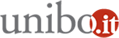# Statistics seminar 2018: Cointegration in functional autoregressive processes

14/11/2018 dalle 14:30 alle 16:30

Dove Dipartimento di Scienze Statistiche - via delle Belle Arti 41 - Aula Seminari - 1° pianoAggiungi l'evento al calendario

Relatore
Massimo Franchi
Università di Roma "La Sapienza"
Dipartimento di Scienze Statistiche

Abstract
This paper defines the class of H valued autoregressive (AR) processes with a unit root of finite type, where H is an infinite dimensional separable Hilbert space, and derives a generalization of the Granger-Johansen Representation Theorem valid for any integration order d=1,2,… . An existence theorem shows that the solution of an AR with a unit root of finite type is necessarily integrated of some finite integer d and displays a common trends representation with a finite number of common stochastic trends of the type of (cumulated) bilateral random walks and an infinite dimensional cointegrating space. A characterization theorem clarifies the connections between the structure of the AR operators and (i) the order of integration, (ii) the structure of the attractor space and the cointegrating space, (iii) the expression of the cointegrating relations, and (iv) the Triangular representation of the process. Except for the fact that the number of cointegrating relations that are integrated of order 0 is infinite, the representation of H-valued ARs with a unit root of finite type coincides with that of usual finite dimensional VARs, which corresponds to the special case H=Rp.

Organizzazione
Alessandra Luati